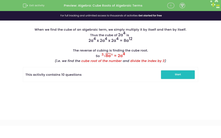# Find the Cube Roots of Algebraic Terms

In this worksheet, students will simplify the cube roots of algebraic terms.Key stage:  KS 3

Curriculum topic:   Algebra

Curriculum subtopic:   Use and Interpret Algebraic Notation

Popular topics:   Algebra worksheets

Difficulty level:#### Worksheet Overview

When we find the cube of an algebraic term, we simply multiply it by itself and then by itself again.

Thus the cube of 2a4 is

2a4 x 2a4 x 2a4 = 8a12

The reverse of cubing is finding the cube root.

So  38a12 = 2a4

This means that we find the cube root of the number and divide the index by 3.

Let's try some questions.

### What is EdPlace?

We're your National Curriculum aligned online education content provider helping each child succeed in English, maths and science from year 1 to GCSE. With an EdPlace account you’ll be able to track and measure progress, helping each child achieve their best. We build confidence and attainment by personalising each child’s learning at a level that suits them.

Get started